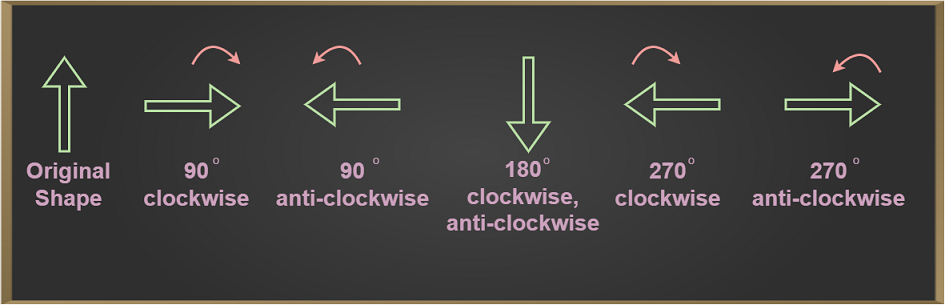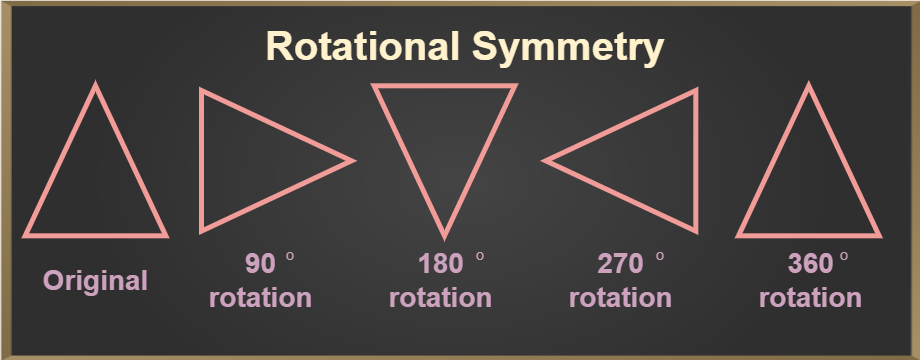Open in App
Not now

# Rotation

• Last Updated : 04 Dec, 2022

In real life, we know that the Earth rotates on its own axis and the moon also rotates on its axis. But what basically rotation is? Also, geometry deals with four basic types of transformations that are Rotation, Reflection, Translation, and Resizing. In this article, we shall read about the fundamental concept of rotation.

## Rotation Definition

Rotation can be defined as the circular motion of an object around its centre or some axis. There can be an infinite number of imaginary lines or axes around which an object rotates. When an object undergoes rotation, all the particles comprising the object move at the same velocity around that axis. In general, rotation is one of the 4 types of transformations. Rotational motion is complex as compared to linear motion.

Rotation may be clockwise or anti-clockwise and an object can be rotated at different angles in rotation. When the rotation is in the clockwise direction, the angle of rotation is considered negative whereas it is considered to be positive in the case of anti-clockwise rotation.

The below image shows an object when rotated through different angles in clockwise and anticlockwise directions.Let us have a look at the rotation formula.

## Rotation Formula

We know that rotation can be done in both clockwise and anti-clockwise directions. In mathematics, rotation refers to the circular motion of a figure around a fixed point, particularly the origin. This leads to a change in the coordinates of the point or figure that is rotated. The rotation can be done around any angle. Let us have a look at the rotation formula for some common angles in both directions when the figure is rotated around the origin.

There is also a general rotation formula when the rotation is not around the origin but around a point Q(α, β) which is as follows:

Let the initial coordinates of the point be (x, y). Then the coordinates of the point after rotation (x’, y’) around point Q are given using

(x’, y’) = {α + (x-α)cosθ – (y-β)sinθ, β + (x-α)sinθ – (y-β)cosθ}

## Rotational Matrix

With the help of a rotation matrix, rotation can be performed in Euclidean space. The matrix rotates a point in an anticlockwise direction by an angle θ and provides the coordinates of the point after the rotation through that angle in the Cartesian Plane. The rotation matrix R can be represented as:This matrix can be then multiplied with a point represented using vector V as follows:## Rotational  Symmetry

Rotational symmetry is the property of the figure due to which its shape remains the same on rotation as compared to the original shape. These figures have an axis of symmetry and are said to possess rotational symmetry. Rotational symmetry can be found using many ways. The easiest way is to start rotating the object from 0° to 360°. If the shape of an object during rotation coincides with its original shape then it is said to possess rotational symmetry at that angle of rotation. For example, a Square possesses rotational symmetry when rotated through angles that are multiples of 90°, a circle possesses rotational symmetry at all angles of rotation whereas a triangle possesses no rotational symmetry.## Solved Examples on Rotational Formula

Example 1: Calculate the coordinates of the point (5, 3) after rotating 90° clockwise.

Solution:

Given, (x, y) = (5, 3)

After rotating the point 90° clockwise the coordinates are given using (y, -x)

Thus, the coordinates of the point after rotation are (3, -5).

Example 2: Calculate the coordinates of the point (3, 4) after rotating 180° anti-clockwise.

Solution:

Given, (x, y) = (3, 4)

After rotating the point 180° anti-clockwise the coordinates are given using (-x, -y)

Thus, the coordinates of the point after rotation are (-3, -4).

Example 3: The coordinates of the point (x, y) after rotating 270° clockwise are (3, 8). What are the actual coordinates of the point?

Solution:

Given, initial coordinates as (x, y)

Final coordinates after rotation are (3, 8). We know that final coordinates after rotating 270° clockwise are given by (-y, x).

Thus (-y, x) = (3, 8)

So (x, y) = (8, -3)

Thus, the actual coordinates of the point are (8, -3).

Example 4: Find the coordinates of the point (1, -6) after rotating 90° anti-clockwise.

Solution:

Given, (x, y) = (1, -6)

After rotating the point 90° clockwise the coordinates are given using (-y, x)

Thus, the coordinates of the point after rotation are (6, 1).

Example 5: Find the coordinates of point (4, 5) when it is rotated 45° anti-clockwise around point Q(5, 5).

Solution:

Given, (x, y) = (4, 5), θ = 45°(positive because the rotation is anti-clockwise), (α, β) = (5, 5)

Using the rotation formula when the point is rotated about a given point:

(x’, y’) = (α + (x – α) cosθ – (y – β) sinθ, β + (x – α) sinθ – (y – β) cosθ)

= (5 + (4 – 5) cos45° – (5 – 5) sin45°, 5 + (4 – 5) sin45° – (5 – 5) cos45°)

= (5 – 1/√2 – 0, 5 – 1/√2 – 0)

Thus, (x’, y’) = (5 – 1/√2, 5 – 1/√2)

## FAQs on Rotation

Question 1: What is meant by rotation?

Rotation is a type of transformation it is defined as the circular motion of an object around a centre or an axis or a fixed point.

Question 2: Write about the 3 types of rotation.

The three types of rotation are:

• Precession
• Nutation
• Intrinsic Rotation

Question 3: Explain the examples of rotation.

Some examples of rotation are:

• Motion of Earth along its axis.
• Motion of a Fan.
• Motion of any globe on its axis.

Question 4: What is an angle of rotation?

Angle of rotation is the amount of rotation measured in degrees.

Question 4: What is the point of rotation?